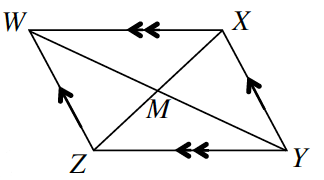### Home > CCG > Chapter 7 > Lesson 7.2.6 > Problem7-108

7-108.What else can you prove about parallelograms? Prove that the diagonals of a parallelogram bisect each other. For example, assuming that quadrilateral $WXYZ$ at right is a parallelogram, prove that $\overline{WM}≅\overline{YM}$ and $\overline{ZM}≅\overline{XM}$.Organize your reasoning in a flowchart. Then record your theorem in your Theorem Toolkit (Lesson 7.2.1A Resource Page).

Start by proving $ΔXWM≅ΔZYM$ by $\text{ASA}≅$. Think about parallel lines and angles while working on this proof.

What reason justifies that $\overline{WM}≅\overline{YM}$ and $\overline{ZM}≅\overline{XM}$?